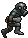Kategori : Python28 Februari 2017 15:39:25

# Python Strftime String Format

Code Meaning Example
`%a` Weekday as locale’s abbreviated name. `Mon`
`%A` Weekday as locale’s full name. `Monday`
`%w` Weekday as a decimal number, where 0 is Sunday and 6 is Saturday. `1`
`%d` Day of the month as a zero-padded decimal number. `30`
`%-d` Day of the month as a decimal number. (Platform specific) `30`
`%b` Month as locale’s abbreviated name. `Sep`
`%B` Month as locale’s full name. `September`
`%m` Month as a zero-padded decimal number. `09`
`%-m` Month as a decimal number. (Platform specific) `9`
`%y` Year without century as a zero-padded decimal number. `13`
`%Y` Year with century as a decimal number. `2013`
`%H` Hour (24-hour clock) as a zero-padded decimal number. `07`
`%-H` Hour (24-hour clock) as a decimal number. (Platform specific) `7`
`%I` Hour (12-hour clock) as a zero-padded decimal number. `07`
`%-I` Hour (12-hour clock) as a decimal number. (Platform specific) `7`
`%p` Locale’s equivalent of either AM or PM. `AM`
`%M` Minute as a zero-padded decimal number. `06`
`%-M` Minute as a decimal number. (Platform specific) `6`
`%S` Second as a zero-padded decimal number. `05`
`%-S` Second as a decimal number. (Platform specific) `5`
`%f` Microsecond as a decimal number, zero-padded on the left. `000000`
`%z` UTC offset in the form +HHMM or -HHMM (empty string if the the object is naive).
`%Z` Time zone name (empty string if the object is naive).
`%j` Day of the year as a zero-padded decimal number. `273`
`%-j` Day of the year as a decimal number. (Platform specific) `273`
`%U` Week number of the year (Sunday as the first day of the week) as a zero padded decimal number. All days in a new year preceding the first Sunday are considered to be in week 0. `39`
`%W` Week number of the year (Monday as the first day of the week) as a decimal number. All days in a new year preceding the first Monday are considered to be in week 0. `39`
`%c` Locale’s appropriate date and time representation. `Mon Sep 30 07:06:05 2013`
`%x` Locale’s appropriate date representation. `09/30/13`
`%X` Locale’s appropriate time representation. `07:06:05`
`%%` A literal '%' character. `%`

Source : http://strftime.org/

Kategori : Python28 Februari 2017 15:39:25

zuka.one